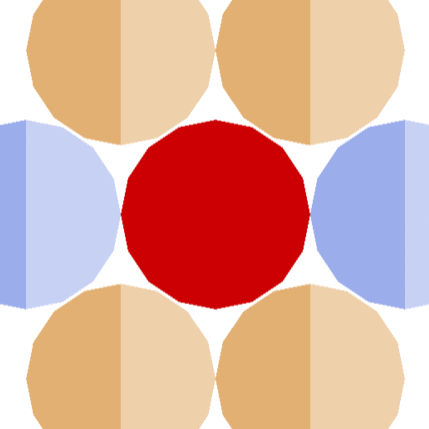# DNA-Dave, the Transcription Translation Robot Educator

DNA-Dave is striving to be the best tool to teach kids molecular biology concepts. The key word is "striving."

IntermediateWork in progressOver 4 days1,201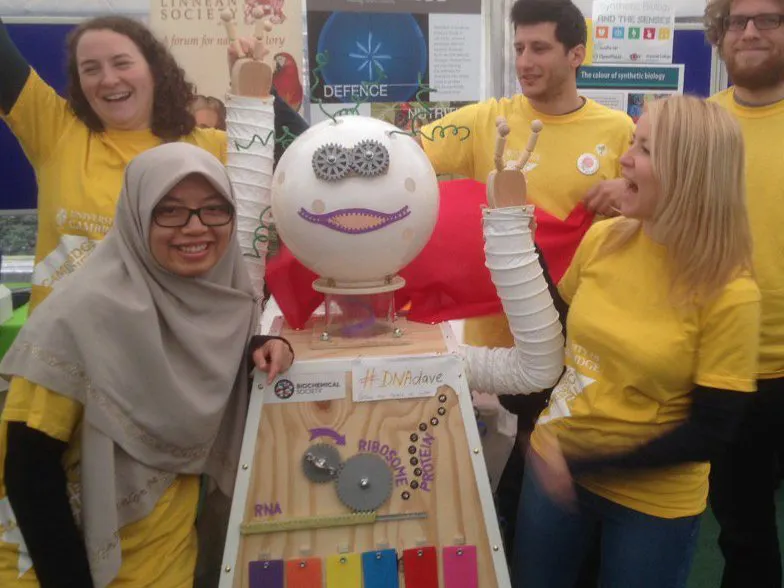## Things used in this project

### Hardware components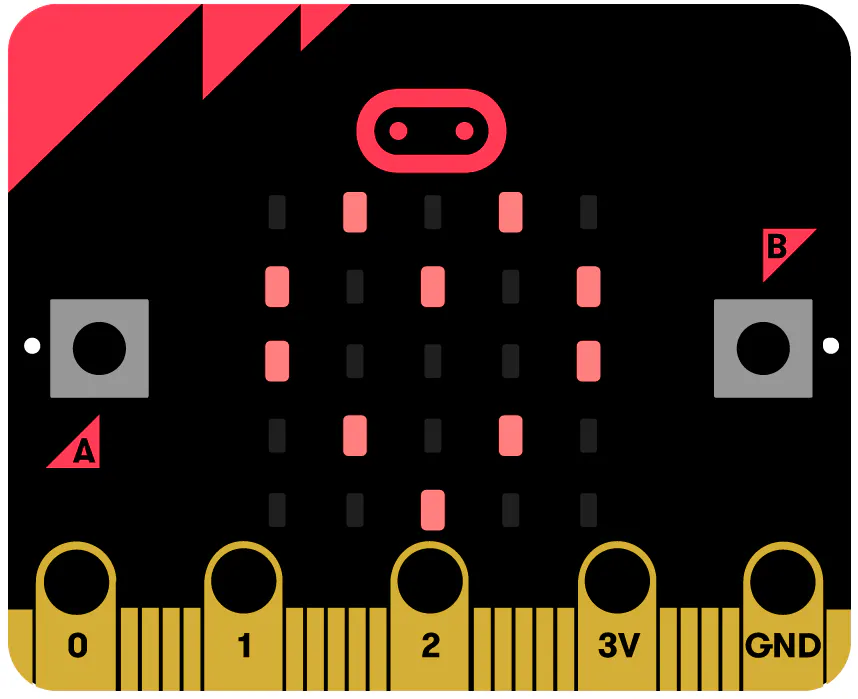BBC micro:bit board
×1
 ElecFreaks octopus:bit
×1
×1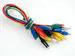Alligator Clips
×1Arduino UNO & Genuino UNO
×1

### Software apps and online servicesMicrosoft MakeCode

## Custom parts and enclosures

eDrawings file of all of DNA Dave's components. We suggest that you download solidworks eDrawings Viewer (which is free!).

## Schematics

### DNA-Dave_Arduino

fritzing schematic file

### DNA-Dave_Arduino_png

schematic png file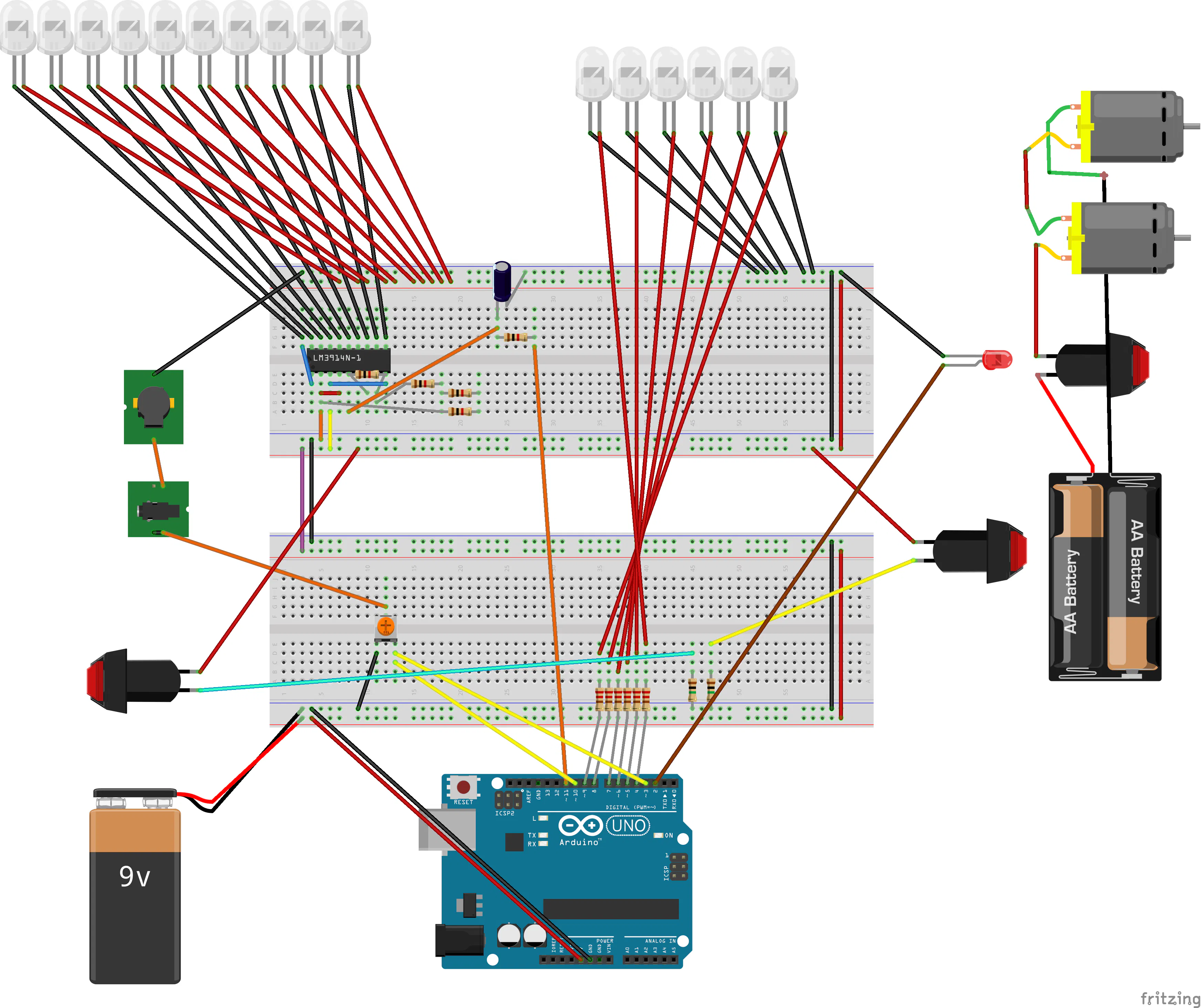### DNA-Dave_microbit

fritzing diagram of microbit version of DNA-Dave. simpler wiring and code than the arduino version

### DNA-Dave_microbit_diagram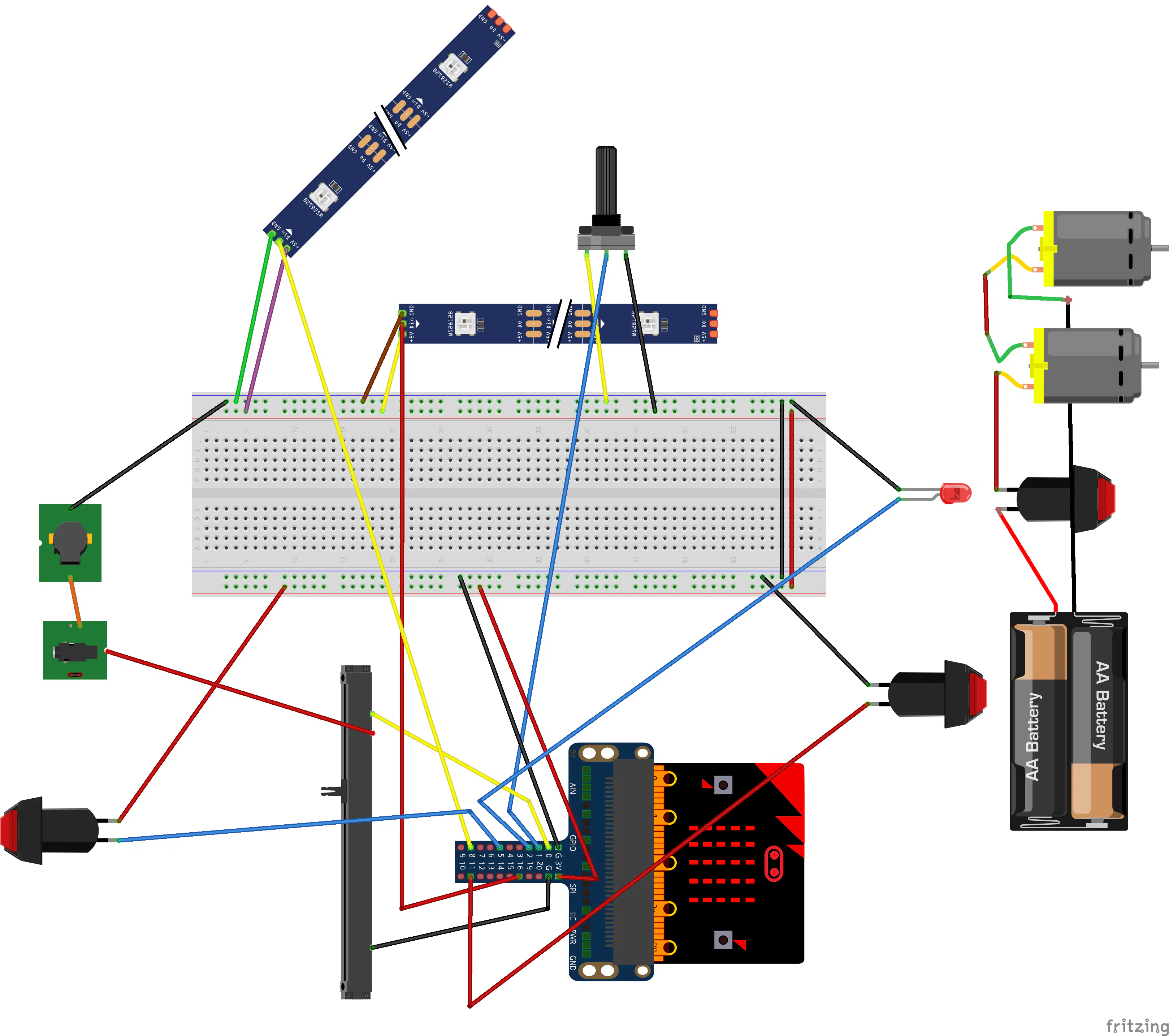## Code

### miniDave_microbit_DNA_translator

JavaScript
this is Javascript MakeCode code for a microbit connected to a strip of individually addressable LEDs, where you can input and visualise a DNA sequence, then translate it into an amino-acid sequence! Just paste it into the Javascript Window of MakeCode online editor. The AminoAcid color scheme is similar to RasMol amino color scheme, itself similar to Shapely scheme.
```function transcribe_and_translate_the_DNA_string () {
RNA_string = []
AminoAcid_string = []
for (let index = 0; index <= DNA_string.length; index++) {
if (DNA_string[index] == "t") {
RNA_string.insertAt(index, "u")
} else {
RNA_string.insertAt(index, DNA_string[index])
}
}
number_of_triplets = RNA_string.length / 3
for (let index2 = 0; index2 <= number_of_triplets; index2++) {
RNA_triplet_position_1 = RNA_string.shift()
RNA_triplet_position_2 = RNA_string.shift()
RNA_triplet_position_3 = RNA_string.shift()
RNA_triplet = "" + RNA_triplet_position_1 + RNA_triplet_position_2 + RNA_triplet_position_3
AminoAcid = Universal_Genetic_Code_squashed[Universal_Genetic_Code_squashed.indexOf(RNA_triplet) + 1]
AminoAcid_string.push(AminoAcid)
}
}
input.onButtonPressed(Button.B, function () {
if (tilt_state == 1) {
DNA_string.push("g")
basic.showLeds(`
. # # # .
# . . . .
# . # # .
# . . # .
. # # # .
`)
} else {
DNA_string.push("a")
basic.showLeds(`
. # # . .
# . . # .
# . . # .
# # # # .
# . . # .
`)
}
})
input.onButtonPressed(Button.A, function () {
if (tilt_state == 0) {
DNA_string.push("t")
basic.showLeds(`
# # # # #
. . # . .
. . # . .
. . # . .
. . # . .
`)
} else {
DNA_string.push("c")
basic.showLeds(`
. # # # .
# . . . .
# . . . .
# . . . .
. # # # .
`)
}
})
input.onGesture(Gesture.Shake, function () {
if (translate_state == 0) {
translate_state = 1
strip.clear()
strip.show()
basic.showString("Protein")
} else {
translate_state = 0
basic.showString("DNA")
strip.clear()
strip.show()
}
})
input.onGesture(Gesture.TiltRight, function () {
tilt_state = 1
basic.showLeds(`
# # . . .
# . # # #
# # # . .
. . # . #
. . # # #
`)
})
input.onButtonPressed(Button.AB, function () {
DNA_string = []
basic.showLeds(`
# . . . #
. # . # .
. . # . .
. # . # .
# . . . #
`)
strip.clear()
})
input.onGesture(Gesture.TiltLeft, function () {
tilt_state = 0
basic.showLeds(`
# # # . .
. # . # .
. # # . #
. . # # #
. . # . #
`)
})
let position = 0
let AminoAcid = ""
let RNA_triplet = ""
let RNA_triplet_position_3 = ""
let RNA_triplet_position_2 = ""
let RNA_triplet_position_1 = ""
let number_of_triplets = 0
let RNA_string: string[] = []
let Universal_Genetic_Code_squashed: string[] = []
let AminoAcid_string: string[] = []
let DNA_string: string[] = []
let translate_state = 0
let tilt_state = 0
let strip: neopixel.Strip = null
strip = neopixel.create(DigitalPin.P0, 40, NeoPixelMode.RGB)
tilt_state = 0
translate_state = 0
DNA_string = []
AminoAcid_string = []
basic.showString("DNA!")
Universal_Genetic_Code_squashed = ["uuu", "phe", "uuc", "phe", "uua", "leu", "uug", "leu", "ucu", "ser", "ucc", "ser", "uca", "ser", "ucg", "ser", "uau", "tyr", "uac", "tyr", "uaa", "stop", "uag", "stop", "ugu", "cys", "ugc", "cys", "uga", "stop", "ugg", "trp", "cuu", "leu", "cuc", "leu", "cua", "leu", "cug", "leu", "ccu", "pro", "ccc", "pro", "cca", "pro", "ccg", "pro", "cau", "his", "cac", "his", "caa", "gln", "cag", "gln", "cgu", "arg", "cgc", "arg", "cga", "arg", "cgg", "arg", "auu", "ile", "auc", "ile", "aua", "ile", "aug", "met", "acu", "thr", "acc", "thr", "aca", "thr", "acg", "thr", "aau", "asn", "aac", "asn", "aaa", "lys", "aag", "lys", "agu", "ser", "agc", "ser", "aga", "arg", "agg", "arg", "guu", "val", "guc", "val", "gua", "val", "gug", "val", "gcu", "ala", "gcc", "ala", "gca", "ala", "gcg", "ala", "gau", "asp", "gac", "asp", "gaa", "glu", "gag", "glu", "ggu", "gly", "ggc", "gly", "gga", "gly", "ggg", "gly"]
basic.forever(function () {
position = -1
if (translate_state == 0) {
for (let deoxyribonucleotide of DNA_string) {
position += 1
if (deoxyribonucleotide == "a") {
strip.setPixelColor(position, neopixel.colors(NeoPixelColors.Blue))
} else if (deoxyribonucleotide == "t") {
strip.setPixelColor(position, neopixel.colors(NeoPixelColors.Green))
} else if (deoxyribonucleotide == "c") {
strip.setPixelColor(position, neopixel.colors(NeoPixelColors.Orange))
} else if (deoxyribonucleotide == "g") {
strip.setPixelColor(position, neopixel.colors(NeoPixelColors.Red))
}
}
} else {
transcribe_and_translate_the_DNA_string()
for (let AminoAcid2 of AminoAcid_string) {
position += 1
if (AminoAcid2 == "ala") {
strip.setPixelColor(position, neopixel.rgb(10, 10, 10))
} else if (AminoAcid2 == "arg") {
strip.setPixelColor(position, neopixel.rgb(40, 10, 235))
} else if (AminoAcid2 == "asn") {
strip.setPixelColor(position, neopixel.rgb(0, 150, 255))
} else if (AminoAcid2 == "asp") {
strip.setPixelColor(position, neopixel.rgb(255, 0, 0))
} else if (AminoAcid2 == "cys") {
strip.setPixelColor(position, neopixel.rgb(255, 200, 0))
} else if (AminoAcid2 == "glu") {
strip.setPixelColor(position, neopixel.rgb(230, 10, 10))
} else if (AminoAcid2 == "gln") {
strip.setPixelColor(position, neopixel.rgb(0, 255, 150))
} else if (AminoAcid2 == "gly") {
strip.setPixelColor(position, neopixel.rgb(235, 235, 235))
} else if (AminoAcid2 == "his") {
strip.setPixelColor(position, neopixel.rgb(255, 0, 255))
} else if (AminoAcid2 == "ile") {
strip.setPixelColor(position, neopixel.rgb(15, 90, 15))
} else if (AminoAcid2 == "leu") {
strip.setPixelColor(position, neopixel.rgb(0, 255, 0))
} else if (AminoAcid2 == "lys") {
strip.setPixelColor(position, neopixel.rgb(0, 0, 255))
} else if (AminoAcid2 == "met") {
strip.setPixelColor(position, neopixel.rgb(200, 255, 5))
} else if (AminoAcid2 == "phe") {
strip.setPixelColor(position, neopixel.rgb(0, 0, 50))
} else if (AminoAcid2 == "pro") {
strip.setPixelColor(position, neopixel.rgb(200, 100, 40))
} else if (AminoAcid2 == "ser") {
strip.setPixelColor(position, neopixel.rgb(255, 100, 0))
} else if (AminoAcid2 == "thr") {
strip.setPixelColor(position, neopixel.rgb(255, 50, 0))
} else if (AminoAcid2 == "trp") {
strip.setPixelColor(position, neopixel.rgb(180, 40, 60))
} else if (AminoAcid2 == "tyr") {
strip.setPixelColor(position, neopixel.rgb(10, 0, 40))
} else if (AminoAcid2 == "val") {
strip.setPixelColor(position, neopixel.rgb(10, 200, 10))
} else if (AminoAcid2 == "stop") {
strip.setPixelColor(position, neopixel.colors(NeoPixelColors.Black))
}
}
}
strip.show()
})
```

### miniDave_microbit_DNA_only_visualiser_simple

JavaScript
This is MakeCode JavaScript code to use with a microbit that is connected to a strip/string of individually addressable LEDs. The user can input a DNA sequence using the microbit and see it in the strip of LEDs! It uses the neopixel extension.
```input.onButtonPressed(Button.A, function () {
if (tilt_state == 0) {
DNA_string.push("t")
basic.showLeds(`
# # # # #
. . # . .
. . # . .
. . # . .
. . # . .
`)
} else {
DNA_string.push("c")
basic.showLeds(`
. # # # .
# . . . .
# . . . .
# . . . .
. # # # .
`)
}
})
input.onButtonPressed(Button.B, function () {
if (tilt_state == 1) {
DNA_string.push("g")
basic.showLeds(`
. # # # .
# . . . .
# . # # .
# . . # .
. # # # .
`)
} else {
DNA_string.push("a")
basic.showLeds(`
. # # . .
# . . # .
# . . # .
# # # # .
# . . # .
`)
}
})
input.onGesture(Gesture.TiltLeft, function () {
tilt_state = 0
basic.showLeds(`
# # # . .
. # . # .
. # # . #
. . # # #
. . # . #
`)
})
input.onGesture(Gesture.TiltRight, function () {
tilt_state = 1
basic.showLeds(`
# # . . .
# . # # #
# # # . .
. . # . #
. . # # #
`)
})
input.onButtonPressed(Button.AB, function () {
DNA_string = []
basic.showLeds(`
# . . . #
. # . # .
. . # . .
. # . # .
# . . . #
`)
strip.clear()
})
let position = 0
let DNA_string: string[] = []
let tilt_state = 0
let strip: neopixel.Strip = null
strip = neopixel.create(DigitalPin.P16, 150, NeoPixelMode.RGB)
tilt_state = 0
DNA_string = []
basic.showString("DNA!")
basic.forever(function () {
position = -1
for (let deoxyribonucleotide of DNA_string) {
position += 1
if (deoxyribonucleotide == "a") {
strip.setPixelColor(position, neopixel.colors(NeoPixelColors.Red))
} else if (deoxyribonucleotide == "t") {
strip.setPixelColor(position, neopixel.colors(NeoPixelColors.Orange))
} else if (deoxyribonucleotide == "c") {
strip.setPixelColor(position, neopixel.colors(NeoPixelColors.Blue))
} else if (deoxyribonucleotide == "g") {
strip.setPixelColor(position, neopixel.colors(NeoPixelColors.Indigo))
}
}
strip.show()
})
```

### DNA-Dave_microbit_javascriptFromMakeCode

JavaScript
goto Makecode website and paste it into the javascript part of a project
```function Glissanto (note1: number, note2: number, rate: number) {
if (note1 < note2) {
i = note1
while (i < note2) {
music.ringTone(i)
control.waitMicros(rate)
i += 2
}
} else {
i = note1
while (i > note2) {
music.ringTone(i)
control.waitMicros(rate)
i += -2
}
}
music.rest(0)
}
function Tremolo (note: number, length: number, rate: number) {
note_duration = length / rate
pauseBetweenNotes = rate * (1 + rate / length)
for (let index = 0; index <= rate; index++) {
music.playTone(note, note_duration)
music.rest(pauseBetweenNotes)
}
music.rest(0)
}
input.onButtonPressed(Button.A, function () {
greenArmpitButtonSound()
control.waitMicros(1000)
})
input.onButtonPressed(Button.B, function () {
redArmpitButtonSound()
control.waitMicros(1000)
})
function success_sound () {
for (let index2 = 0; index2 <= Math.randomRange(1, 6); index2++) {
for (let index22 = 0; index22 <= 1000; index22++) {
music.ringTone(1000 + index22)
control.waitMicros(300)
}
}
music.rest(music.beat(BeatFraction.Whole))
music.stopMelody(MelodyStopOptions.All)
}
function angry_Dave_sound () {
for (let index3 = 0; index3 <= 4; index3++) {
for (let index23 = 0; index23 <= 1000; index23++) {
music.ringTone(1000 - index23)
control.waitMicros(300)
}
}
music.rest(music.beat(BeatFraction.Whole))
music.stopMelody(MelodyStopOptions.All)
}
function dispense_protein () {
which_protein = Math.randomRange(0, strip2.length() - 1)
strip2.setPixelColor(which_protein, neopixel.rgb(Math.randomRange(0, 255), Math.randomRange(0, 255), Math.randomRange(0, 255)))
strip2.setPixelColor(Math.abs((which_protein - 1) % strip2.length()), neopixel.rgb(Math.randomRange(0, 255), Math.randomRange(0, 255), Math.randomRange(0, 255)))
strip2.show()
for (let index4 = 0; index4 <= 9 * strip2.length() - 1; index4++) {
strip2.rotate(1)
strip2.show()
music.ringTone(index4 * index4)
control.waitMicros(index4 * 7000)
}
strip2.clear()
strip2.setPixelColor(which_protein, neopixel.colors(NeoPixelColors.Green))
strip2.show()
success_sound()
control.waitMicros(6000000)
strip.clear()
strip.show()
control.waitMicros(2000000)
strip2.clear()
strip2.show()
}
function redArmpitButtonSound () {
rand = Math.randomRange(1, 4)
if (rand == 1) {
Glissanto(784, 392, 0)
Glissanto(392, 784, 0)
control.waitMicros(100000)
Glissanto(784, 392, 0)
Glissanto(392, 784, 0)
}
if (rand == 2) {
Glissanto(1400, 1000, 0)
Glissanto(1200, 800, 0)
Glissanto(1000, 6000, 0)
control.waitMicros(300000)
Tremolo(440, 30, 3)
control.waitMicros(100000)
Tremolo(659, 30, 12)
}
if (rand > 2) {
Glissanto(784, 392, 0)
control.waitMicros(300000)
Glissanto(784, 392, 0)
Glissanto(784, 196, 0)
}
}
function greenArmpitButtonSound () {
rand = Math.randomRange(1, 5)
if (rand == 1) {
Glissanto(784, 392, 0)
control.waitMicros(300000)
Glissanto(880, 220, 0)
control.waitMicros(100000)
Tremolo(175, 30, 3)
control.waitMicros(100000)
Tremolo(131, 30, 3)
control.waitMicros(100000)
Glissanto(131, 523, 0)
Glissanto(988, 392, 0)
}
if (rand == 2) {
Glissanto(500, 2000, 0)
control.waitMicros(500000)
Tremolo(800, 30, 3)
control.waitMicros(100000)
Tremolo(600, 30, 3)
control.waitMicros(100000)
Glissanto(300, 1700, 0)
Glissanto(600, 3000, 0)
}
if (rand == 3) {
Glissanto(6000, 200, 0)
control.waitMicros(500000)
Tremolo(1000, 30, 3)
control.waitMicros(100000)
Tremolo(600, 30, 3)
control.waitMicros(100000)
Tremolo(800, 30, 3)
control.waitMicros(100000)
Tremolo(400, 30, 3)
}
if (rand == 4) {
Glissanto(6000, 5500, 100)
control.waitMicros(100000)
Glissanto(6000, 5500, 100)
control.waitMicros(300000)
Glissanto(6000, 5500, 100)
control.waitMicros(100000)
Tremolo(1000, 30, 3)
control.waitMicros(10000)
Tremolo(600, 30, 3)
control.waitMicros(10000)
Tremolo(800, 30, 3)
control.waitMicros(10000)
Tremolo(400, 30, 3)
}
if (rand == 5) {
Glissanto(294, 2000, 0)
control.waitMicros(500000)
Tremolo(1760, 30, 3)
control.waitMicros(100000)
Tremolo(1396, 30, 3)
control.waitMicros(100000)
Glissanto(5000, 2500, 0)
Glissanto(5000, 2500, 0)
}
}
let which_LED = 0
let cog_average_before = 0
let cog_average = 0
let Dave_state = 0
let cog_voltage = 0
let rand = 0
let which_protein = 0
let pauseBetweenNotes = 0
let note_duration = 0
let i = 0
let strip2: neopixel.Strip = null
let strip: neopixel.Strip = null
strip = neopixel.create(DigitalPin.P8, 10, NeoPixelMode.RGB)
strip2 = neopixel.create(DigitalPin.P16, 6, NeoPixelMode.RGB)
let increment = 20
let dispensed_protein = 1
basic.forever(function () {
control.waitMicros(1000)
if (Dave_state == 0) {
strip.clear()
strip.show()
cog_average = 0
pins.digitalWritePin(DigitalPin.P2, 1)
if (cog_voltage < 20) {
pins.digitalWritePin(DigitalPin.P2, 0)
strip.clear()
strip2.clear()
strip.show()
strip2.show()
Dave_state = 1
cog_average_before = 20
}
} else if (Dave_state == 1) {
cog_average += (cog_voltage - cog_average) * 0.7
which_LED = Math.round(Math.map(cog_average, 0, 1023, 0, strip.length() + 2))
for (let index5 = 0; index5 <= which_LED; index5++) {
strip.setPixelColor(index5, neopixel.rgb(Math.randomRange(0, 255), Math.randomRange(0, 255), Math.randomRange(0, 255)))
}
strip.show()
if (cog_average > cog_average_before + increment) {
cog_average_before = cog_average
} else if (cog_average < cog_average_before - increment) {
Dave_state = 2
}
if (cog_average > 740) {
Dave_state = 3
}
} else if (Dave_state == 2) {
angry_Dave_sound()
Dave_state = 0
} else if (Dave_state == 3) {
success_sound()
dispense_protein()
Dave_state = 0
strip.clear()
strip.show()
}
})
```

### DNA-Dave_Arduino

This is the code that runs on the arduino version of DNA-Dave

## Credits

### Ioannis Tamvakis

1 project • 1 follower

### sami.s

0 projects • 0 followers